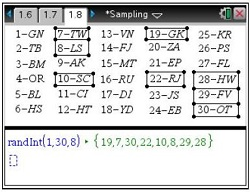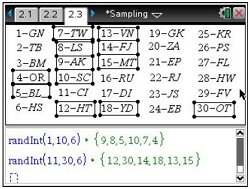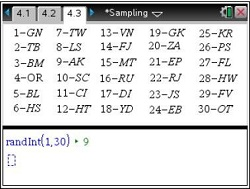# Activities

••• ##### Subject Area

• Math: Statistics: Data Analysis
• Math: Statistics: Statistical Inference

• ##### Author9-12

25 Minutes

• ##### Device
•TI-Nspire™ CX/CX II
•TI-Nspire™ CX CAS/CX II CAS
• TI-Nspire™
• TI-Nspire™ CAS
• ##### Software

TI-Nspire™
TI-Nspire™ CAS

3.2

Sampling

#### Activity Overview

Students learn about each of the four types of random sampling methods and use the randInt command to find each kind of sample from a given population.

#### Key Steps

•Students explore random sampling in problem 1. They discuss populations, samples, and what it means for a sample to be representative of the population.

Students move on and learn four types of probability samples. They will use the random integer command to randomly selected 8 people for a sample.

•Students discuss a stratified random sample, often just called a stratified sample.

They will find a random sample of 12 people from a list, where half are men and half are women. After discussing why this method would produce a disproportionate sample, students will use the random integer command to select a proportionate sample.

•Students will also use the random integer command to simulate selecting a sample using cluster sampling and systematic sampling. At the end of this activity, students distinguish between the types of probability sampling methods.